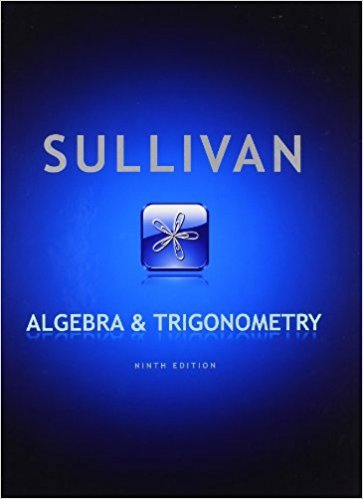×
×

# Solutions for Chapter 12.5: Algebra and Trigonometry 9th Edition## Full solutions for Algebra and Trigonometry | 9th Edition

ISBN: 9780321716569Solutions for Chapter 12.5

Solutions for Chapter 12.5
4 5 0 351 Reviews
24
5
##### ISBN: 9780321716569

Algebra and Trigonometry was written by and is associated to the ISBN: 9780321716569. Since 46 problems in chapter 12.5 have been answered, more than 61875 students have viewed full step-by-step solutions from this chapter. Chapter 12.5 includes 46 full step-by-step solutions. This textbook survival guide was created for the textbook: Algebra and Trigonometry, edition: 9. This expansive textbook survival guide covers the following chapters and their solutions.

Key Math Terms and definitions covered in this textbook
• Affine transformation

Tv = Av + Vo = linear transformation plus shift.

• Associative Law (AB)C = A(BC).

Parentheses can be removed to leave ABC.

• Cholesky factorization

A = CTC = (L.J]))(L.J]))T for positive definite A.

• Companion matrix.

Put CI, ... ,Cn in row n and put n - 1 ones just above the main diagonal. Then det(A - AI) = ±(CI + c2A + C3A 2 + .•. + cnA n-l - An).

• Complex conjugate

z = a - ib for any complex number z = a + ib. Then zz = Iz12.

• Diagonal matrix D.

dij = 0 if i #- j. Block-diagonal: zero outside square blocks Du.

• Factorization

A = L U. If elimination takes A to U without row exchanges, then the lower triangular L with multipliers eij (and eii = 1) brings U back to A.

• Full row rank r = m.

Independent rows, at least one solution to Ax = b, column space is all of Rm. Full rank means full column rank or full row rank.

• Hankel matrix H.

Constant along each antidiagonal; hij depends on i + j.

• Iterative method.

A sequence of steps intended to approach the desired solution.

• Length II x II.

Square root of x T x (Pythagoras in n dimensions).

• Multiplication Ax

= Xl (column 1) + ... + xn(column n) = combination of columns.

• Network.

A directed graph that has constants Cl, ... , Cm associated with the edges.

• Normal equation AT Ax = ATb.

Gives the least squares solution to Ax = b if A has full rank n (independent columns). The equation says that (columns of A)·(b - Ax) = o.

• Partial pivoting.

In each column, choose the largest available pivot to control roundoff; all multipliers have leij I < 1. See condition number.

• Rotation matrix

R = [~ CS ] rotates the plane by () and R- 1 = RT rotates back by -(). Eigenvalues are eiO and e-iO , eigenvectors are (1, ±i). c, s = cos (), sin ().

• Stiffness matrix

If x gives the movements of the nodes, K x gives the internal forces. K = ATe A where C has spring constants from Hooke's Law and Ax = stretching.

• Toeplitz matrix.

Constant down each diagonal = time-invariant (shift-invariant) filter.

• Vandermonde matrix V.

V c = b gives coefficients of p(x) = Co + ... + Cn_IXn- 1 with P(Xi) = bi. Vij = (Xi)j-I and det V = product of (Xk - Xi) for k > i.

• Vector space V.

Set of vectors such that all combinations cv + d w remain within V. Eight required rules are given in Section 3.1 for scalars c, d and vectors v, w.

×